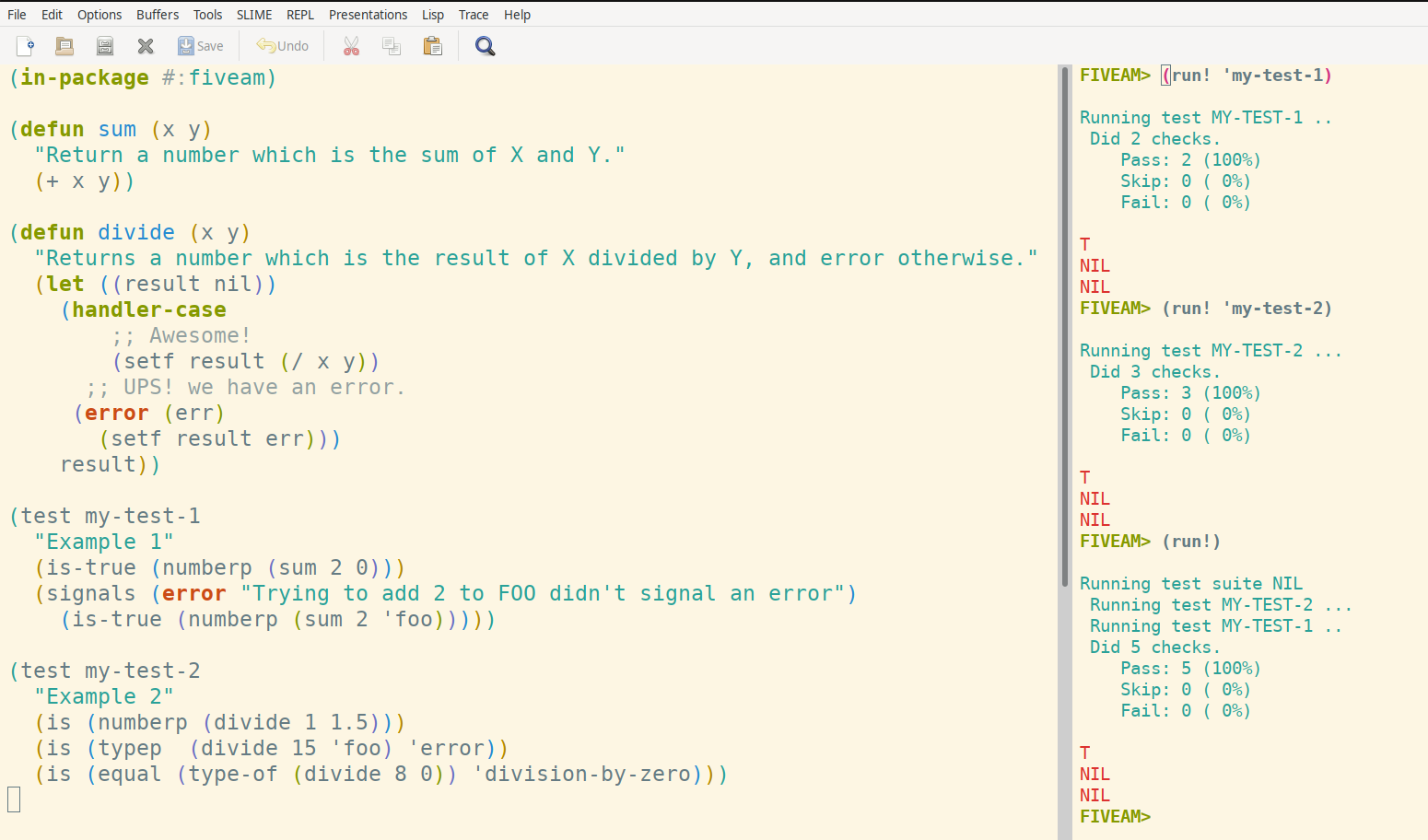informaticsEn 2021-10-15 10:51:58
122

# Are you looking for a test framework in Common Lisp?

FiveAM is a Test Framework whose goal is to be as simple as possible.

https://github.com/lispci/fiveamNow We are going to define two functions

(defun sum (x y)
"Return a number which is the sum of X and Y."
(+ x y))

and

(defun divide (x y)
"Returns a number which is the result of X divided by Y, and error otherwise."
(let ((result nil))
(handler-case
;; Awesome!
(setf result (/ x y))
;; UPS! we have an error.
(error (err)
(setf result err)))
result))

Define test cases

(test my-test-1
"Example 1"
(is-true (numberp (sum 2 0)))
(signals (error "Trying to add 2 to FOO didn't signal an error")
(is-true (numberp (sum 2 'foo)))))

(test my-test-2
"Example 2"
(is (numberp (divide 1 1.5)))
(is (typep  (divide 15 'foo) 'error))
(is (equal (type-of (divide 8 0)) 'division-by-zero)))

Run tests

FIVEAM> (run! 'my-test-1)

Running test MY-TEST-1 ..

Did 2 checks.

Pass: 2 (100%)

Skip: 0 ( 0%)

Fail: 0 ( 0%)

T

NIL

NIL

FIVEAM> (run! 'my-test-2)

Running test MY-TEST-2 ...
Did 3 checks.
Pass: 3 (100%)
Skip: 0 ( 0%)
Fail: 0 ( 0%)

T
NIL
NIL
FIVEAM>